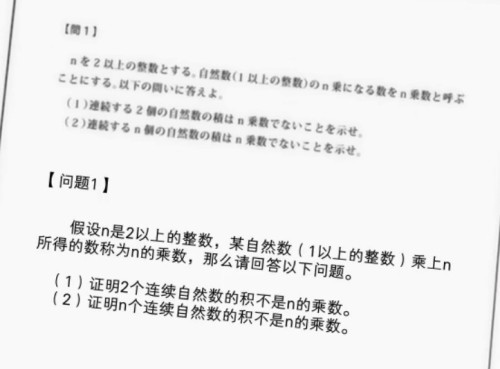# 看新番学知识系列 No.6：《伪恋》第四集出现的题目怎么解？：学霸是一种阿宅不敢企及的稀有生物，但假如倒贴的妹子是香菜，或许阿宅也能“重金之下必有勇夫”吧？那么《伪恋》第四集里几位主角的学习会上的那道题目，应该怎么样证明才能避免被千棘鄙视，获得小咲的赞赏呢？

（提示：字幕组翻译错了重要的一点，这里n乘数特指n次幂，也就是a^n。换而言之，求证n个连续自然数的积，不可能表达为m^n的形式。不准备亲自尝试的人，就接着看吧。）

：或许由于大部分动画里出现的题目都过于简单，导致我国观众对日本的高等教育水平可能有不正确的猜测吧？其实日本高中数学和欧洲相近，都会在大学前做不少微积分相关的学习（至少达到国内大一水准），所以在很大程度上，比中国的高中数学内容还多些。

• m与m+1互素。
• 据此，m和m + 1都必须自身也是n次幂，否则积不可能是n次幂。
• 利用反证法证伪。

• 假如k到(k+n-1)的积等于m^n，则m^n必须大于k^n，小于(k+n-1)^n。
• 换而言之，m>k AND m < k+n-1，我们可将它表达为k + i，也就是求证积可表达为(k+i)^n。
• 但k到(k+n-1)的积包含k+i与k+i+1，而我们已知k+i与k+i+1互素，(k+i)^n的约数不可能包含k+i+1。反证完成。

QED.

“看新番学知识”系列是尝试从ACG作品中寻找有趣知识的栏目，提供短小精悍的非弹幕文化资讯供旅客们参考。## Author: 店长

The Master of BitInn

## 8 thoughts on “看新番学知识系列 No.6：《伪恋》第四集出现的题目怎么解？”

1.xX叉叉Xx says:

学知识自豪啊，这满满的近世代数feel。

2.null says:

依然看不懂怎么办。。

3.YUKI.N says:

还只是第一题，真有趣。

4.undefined says:

首问证明要点的第二点写的稍微有点问题。
要想乘积是n次幂，m和m + 1不一定自身非要是n次幂，完全可以m=a^(n-x)*b^(n-y)…，m+1=a^x*b^y….这种形式。
不过还是逃不过互质

5.undefined says:

额，看漏了“据此”，以第一点做前提的话，第二点就没问题了。但第二点到第三点跳跃太大了。在这里稍微补一下
设m=a^n，m+1=b^n，因m+1>m>=1，故b>a>=1
m+1-m=b^n-a^n=1
b^n-a^n=(b-a)[b^(n-1)+b^(n-2)*a+…+b*a^(n-2)+a^(n-1)]=1
又因b-a和b^(n-1)+b^(n-2)*a+…+b*a^(n-2)+a^(n-1)都是大于0的整数，所以只能是
b-a=b^(n-1)+b^(n-2)*a+…+b*a^(n-2)+a^(n-1)=1
然后把b=a+1代入b^(n-1)+b^(n-2)*a+…+b*a^(n-2)+a^(n-1)，得到
(a+1)^(n-1)+(a+1)^(n-2)*a+…+(a+1)*a^(n-2)+a^(n-1)，每一项都>=1
所以(a+1)^(n-1)+(a+1)^(n-2)*a+…+(a+1)*a^(n-2)+a^(n-1)>=n
与前面的=1矛盾

6.FormerA says:

数学已经彻底还给老师了……

7.nopass says:

一大波数论即视感袭来……

8.はる says:

初等数论里的玩意